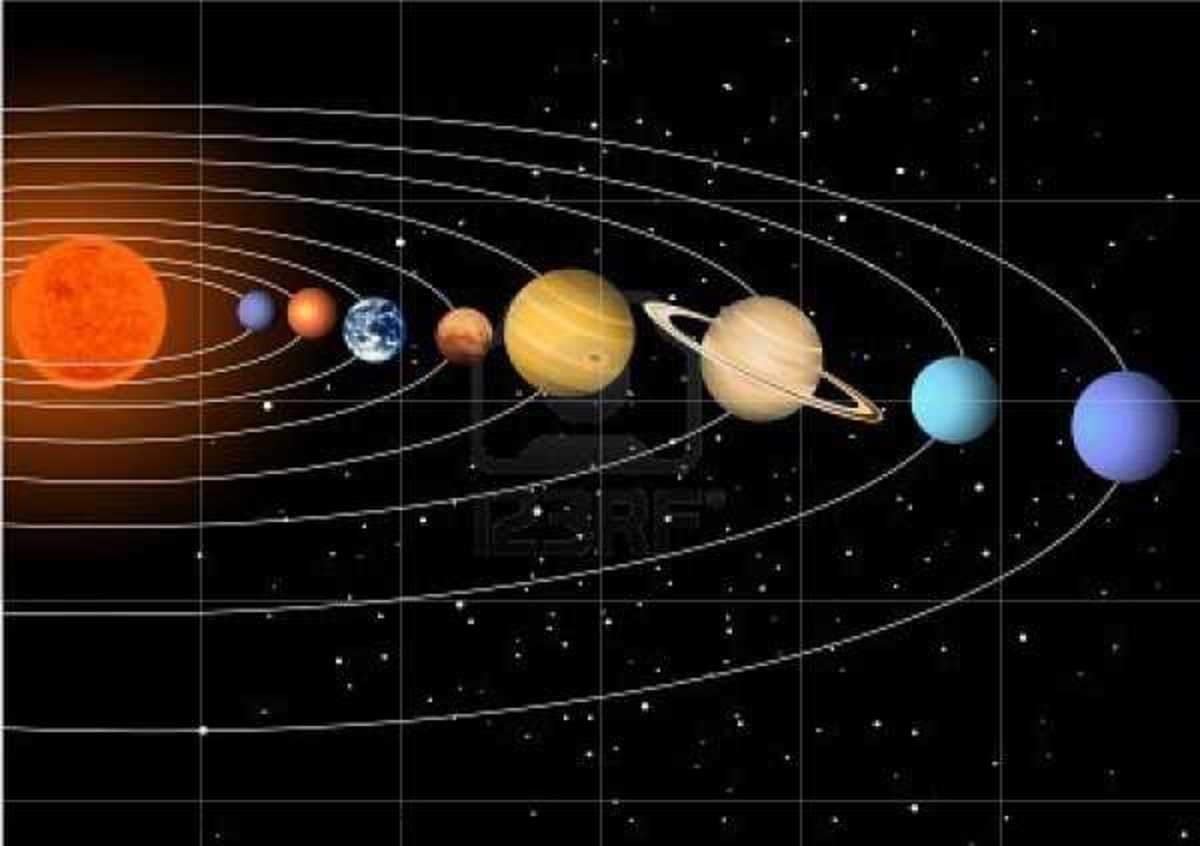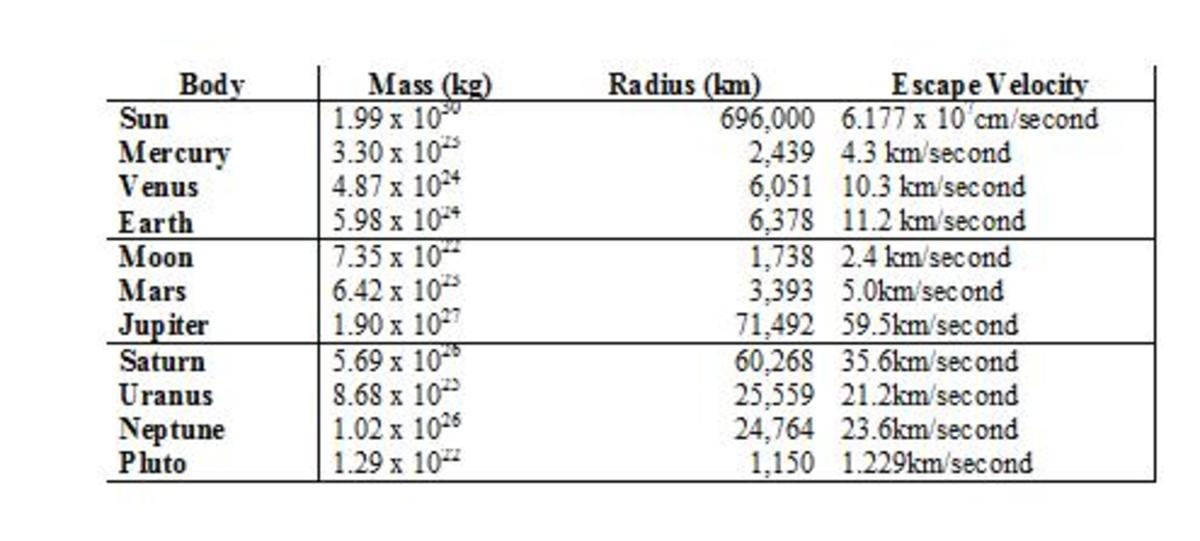# Escape Velocity and the Solar SystemEscape velocity can be determined for the sun, all of the planets within our solar system, and all of their moons. 123RF

## By Joan Whetzel

Ever since the United States began its space program in the 1950s, the National Aeronautics and Space Administration program has expanded to include rockets flying men to the moon, orbital satellites, space stations and probes touring the solar system to see what spring is like on Jupiter and Mars. But none of this would have been possible without first understanding the physics of escape velocity.

Newton’s Law of Gravitation

One of Sir Isaac Newton's discoveries play into getting sending rockets into space. The first is Newton's Universal Law of Gravitation, which describe gravitation as "the force (F) of gravity between two bodies." That force of gravity is equal to both masses (m1 = the mass of the planet, m2 = the mass of the person or object being sent into space) multiplied together, then divided by the distance between (r = the radius of the planet, squared) them squared. That number is then multiplied by the universal gravitational constant (G). The equation looks like this.

F = G (m1 x m2) / r2

Basically what it's saying is that the force of gravity is what attracts the two masses to each other, and the planet or sun, which has the heavier mass and has the gravity working on its side, keeps the object or person with the lesser mass attracted to it. That gravitational force varies, depending on the of the planet or sun and the mass of the of the object to be launched into space as well as the square of the distance between the planet and the object to be launched. Since the gravitational attraction for the sun is different than that of each of the planets of the solar system, and different than each of their moons, then by extension, the escape velocity for the sun will be different from that of each planet and each of their moons.This table lists the mass, radius and escape velocity fo the sun, each of the planets, and the Earth's moon.Joan Whetzel

Escape Velocity Explained

Escape velocity can be defined as the requisite speed for overcoming the gravitational pull of a sun, planet or moon. Imagine a rocket being launched into space, for example. The force of gravity diminishes as the rocket climbs higher into the Earth's atmosphere, which requires less and less force on the part of rocket's propulsion systems, until the rocket finally breaks through the outer atmosphere of the Earth and is propelled into space.

For Earth, then, the escape velocity comes to 6.96 miles per second (11.2 kilometers per second). Frequently, the escape velocity is rounded up to 7 miles per second, which works out to approximately 34 times the speed of sound. While the mass of the object to be launched doesn't affect its escape velocity, it does affect the amount of force, or energy, required to launch it off the ground and to send it into space and beyond the force of gravity.

The amount of force it takes to launch that rocket into the air, beyond the point at which it would fall back to Earth - the point at which the pull of gravity no longer has an effect - is known as Earth's escape velocity (Ve). The equation for this describes escape velocity as twice the gravitational constant (G) multiplied by the mass (M) of the planet, moon, or star. That product is divided by the radius (r) of the planet, moon or star (the distance from the core of the planet, moon or star) and that quotient is then squared. The equation looks something like this:

Ve = [(2GM) / r]2

This equation is based on a planet, moon or star that is uniformly spherical and a projectile body that with a mass that is much smaller than that of the planet. It also does not take into account the rotational effect of the Earth (planet, moon, star) or friction caused by the atmosphere, which would tend to slow the rocket down. Of course, since rockets have their own engines and fuel supplies, they don't actually have to reach escape velocity. They can continually fire their engines until the break through the gravitational pull. The escape velocity equation describes a projectile being launched from the ground, where the only force propelling it is on the ground. No additional force is added during the projectile's flight through the atmosphere.

Escape Velocity Vs. Orbital Velocity

Escape velocity is an entirely different animal than orbital velocity. Orbital velocity is the speed required to keep an object (e.g. a satellite) in orbit around a planet, moon, or star, which means that the object is still being influenced by the pull of gravity. It will still be pulled back to the surface of the planet, moon or star unless it maintains this speed (the orbital velocity).

Escape Velocity in the Solar System

Using this math, the escape velocity for the sun, the planets and some of the moons in our solar system can be calculated Provided you have an asbestos suit for visiting the sun, you could escape the sun's gravitational pull by knowing the its mass (1.99x1030kg) and radius (696,000 km). The same goes for any of the planets and moons in the solar system. You can’t escape the math, only the pull of gravity

Bibliography

Scroll to Continue

University of Tennessee, Knoxville. Sir Isaac Newton: The Universal Law of Gravitation.

Piclover, Clifford a. The Physics Book. New York: Sterling Publishing, 2011.

## Escape Velocity Gravity Well

François Lievens on July 26, 2013:

François Lievens

The equation for the escape velocity is wrong!

It's not the square of 2GM/r but the square root

Daffy Duck from Cornelius, Oregon on February 18, 2012:

It seems so simple when the equation is explained, yet I'll forget all in a week. :)

Joan Whetzel (author) on February 18, 2012:

Thanks Neils, I will check it out.

neils on February 17, 2012:

you should check out this site it gives every explanation in very simple language www.scimad.com

neils on February 17, 2012:

you should check out this site it gives every explanation in very simple language